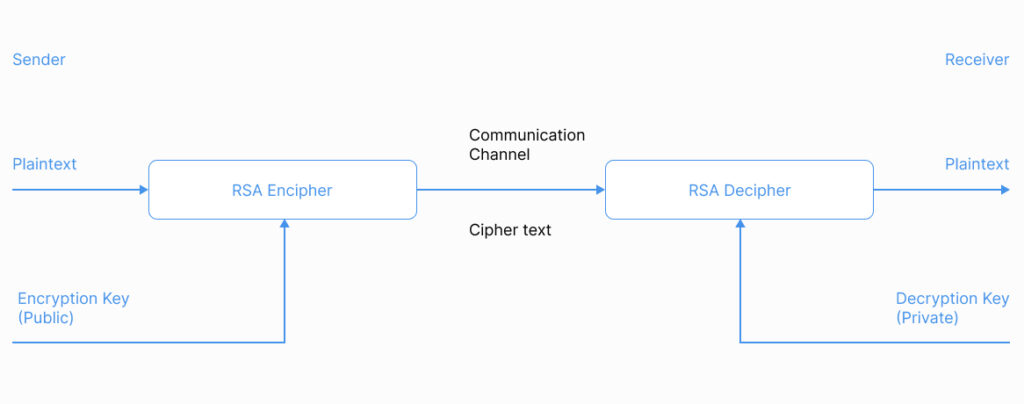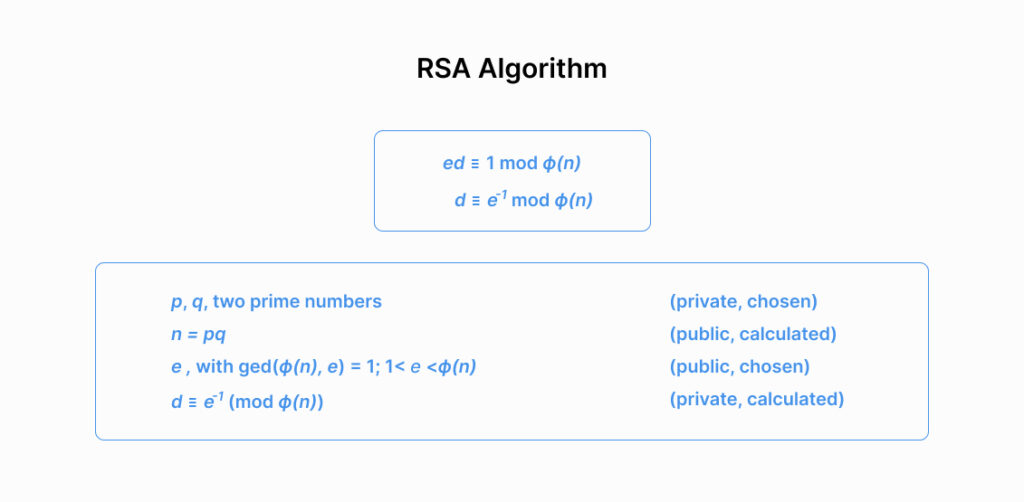• PRODUCTS

SOLUTIONS

• Blog
• Contact
What Is RSA Algorithm (Rivest-Shamir-Adleman) and How Does it Work

DATE:

FEBRUARY 27 2023

AUTHOR:

# RSA Algorithm in Cryptography

The Rivest-Shamir-Adleman cryptographic algorithm RSA is one of the most widely used asymmetric encryption algorithms today. In nearly 50 years, it has received many uses without significant changes. What is RSA and how does it work?

## What Is the RSA Algorithm (Rivest-Shamir-Adleman)?

RSA is an acronym for the inventors’ names Rivest, Shamir, and Adleman. It is a public-key cryptographic algorithm based on the computational complexity of the large prime factorization problem.

The idea of an asymmetric public/private key cryptosystem is attributed to Whitfield Diffie and Martin Hellman who published the concept in 1976. They also introduced RSA signature verification and tried to apply number theory. Their formulation used a shared secret key created by exponentializing some number modulo a prime number.

The RSA cryptosystem was the first system suitable for both encryption and digital signatures. Currently, RSA public key asymmetric encryption is used by most products in the information security market including PGP, S/MIME, TLS/SSL, IPSEC/IKE and others to provide authentication and encryption on the Internet and in private networks.

It is important to note that it has not been proven that the RSA security depends entirely on the problem of finding prime factors of a large number. However, all other ways of finding turned out to be equivalent in cost to the factorization problem. However, so far there has not been such an algorithm found and RSA withstood a huge number of opening attempts.

## Why Is the RSA Algorithm Used?

The RSA is one of the best-known trap door functions in cryptography. In theoretical computer science, a trapdoor function is a function that is easy to compute in one direction, and difficult to compute in the opposite direction without special information, called the “trapdoor”.

In the RSA encryption algorithm for encryption, the operation of exponentiation modulo a large number is used. To decrypt (reverse operation) in a reasonable time, you must be able to calculate the Euler function of a given large number for which you need to know the decomposition of the number into prime factors.

In public key cryptography, each participant has both a public key and a private key. Each key consists of a pair of integers. Participants create their own public and private key. Each of them keeps the private key secret and the public keys can be shared with anyone or even published.

The cryptographic strength of the algorithm is based on the complexity of factoring large numbers namely on the exceptional difficulty of the task for determining the secret key based on the public one, since this will require solving the problem of the existence of integer divisors. The most cryptographically strong systems use 2048-bit and larger numbers.

The RSA is used for digital signing and symmetric key exchange, but it always requires the public RSA key exchange beforehand unlike the Diffie-Hellman exponential key exchange that allows to securely exchange the secret key. Furthermore, it is considered to be safer to the side channel attacks but requires more resources.## How Does the RSA Algorithm Work?

RSA has two uses.

The first is encryption when Alice publishes her public key and Bob knowing it can encrypt a message that only Alice can read by decrypting it with her private key.

The second is a digital signature which allows Alice to sign a message with her private key so that everyone can verify the signature with her public key. Both algorithms differ in minor details so we will simply call them RSA.

To start working with RSA, Alice needs to choose two primes p and q which together form a group of numbers modulo n = p*q. Then Alice needs to choose a public exponent e and a secret d such that ed = 1(mod(p-1)(q-1)). Essentially e and d must be coprime.

Once these options have been chosen in which Bob can then send a message M in ciphertext form to Alice by computing C=M^e (mod(n)). Alice can then decrypt the message by computing M=C^d(mod(n)).

Digital signature is exactly the opposite. If Alice wants to sign a message, she computes a signature S=M^d(mod(n)), which Bob can verify that the message M = S^e(mod(n)).

Since RSA keys generation occurs much less frequently than operations that implement encryption and decryption as well as the creation and verification of a digital signature, the task of calculating a=b^c(mod (n)) is the main computational complexity. This problem can be solved using the fast exponentiation algorithm. This algorithm computing m^e(mod(n)) requires O*(ln e) modulo multiplications.

Let’s look at an RSA example with numbers for key pair generation:

1. Select primes: p=17 & q=11
2. Compute n = pq =17*11=187
3. Compute Euler’s totient function: ø(n)=(p–1)(q-1)=16*10=160
4. Select e using greatest common divisor: gcd(e,160)=1; choose e=7
5. Determine d using Extended Euclid’s algorithm: d*e=1 mod 160 and d < 160, value is d=23 since 23*7=161= 1*160+1
1. Publish public key PU={7,187}
2. Keep secret private key PR={23,187}## How Is RSA Secure?

There is a trend to switch to the safer (Elliptic Curve) Diffie-Hellman key that exchanges from the RSA, but the RSA encryption algorithm is still considered secure. However, when implementing, developers often make mistakes that can lead to hacking.

The RSA algorithm assumes that the large number N is two primes p and q. At the same time, it is difficult to do the decomposition of N into prime factors without knowing p and q. Often, instead of generating a truly random prime number, developers try to generate numbers of a particular shape. It almost always ends badly.

If p or q are ever reused in other RSA modules, then both factors can be easily computed using the GCD algorithm. Bad random number generators are often subject to this attack. It is also worth noting that if p and q share approximately half of their most significant bits, then N can be computed using Fermat’s method.

Using a large RSA private key means more time spent decrypting and signing messages. Therefore, many choose a small d. An attacker can recover the private key when d is less than the 4th root of N.

## Examples of the RSA Encryption Algorithm

As noted earlier, the RSA cryptography is used to protect software and in digital signature schemes. In addition, it is used in the open PGP encryption system and other encryption systems: DarkCryptTC and the xdc format. One of the most common uses of RSA today is the use of RSA as one of the TLS/SSL protocol algorithms. This protocol ensures the protection of data transmission on the Internet.

Asymmetric cryptographic protocols require significantly more time for the encryption and decryption operation compared to symmetric key cryptography. Therefore, messages are usually encrypted using faster symmetric algorithms with a random session key. Examples of such protocols are AES, IDEA, Serpent, Twofish. And with the help of RSA, only this session key is encrypted. Thus, a hybrid cryptosystem is implemented. It combines the benefits of both asymmetric and symmetric encryption and reduces the risks of each.

RSA Key Exchange is also used for the Secure Shell (SSH) Transport Layer Protocol for authentication of the servers. Its applications are mostly remote login and command-line execution. SSH key pairs are used for automating logins, single sign-on, and for authenticating hosts. Using key based logins with ssh is considered more secure than using plain password logins.

The main advantage of the RSA public key encryption algorithm is that having a public key and knowing the encryption algorithm, it is impossible to repeat the encrypted message. It is very easy to share the public key for it, it can be sent or published in a plain text. Over the years, this algorithm has remained safe and reliable. Therefore, it received another advantage – it is widely used in hardware and software products.

In the hardware application, the RSA algorithm is used in secure phones, on Ethernet network cards, on smart cards, and is widely used in cryptographic equipment. The algorithm is included in all major protocols for secure Internet communications, including S/MIME SSL and S/WAN.

To secure the Internet communications, the RSA is used In X.509 public key certificates issued X.509 certificates issued and managed by Certificate Authorities CA that are used in many Internet protocols, including TLS/SSL secure protocol for HTTPS connections when browsing the web.

RSA is also widely used in many institutions such as government agencies, most corporations, government laboratories and universities.

## Conclusion

RSA is one of the most widely used asymmetric cryptographic algorithms today. It has been one of the most popular and secure tools for encryption and digital signature for decades. However, it is extremely important to correctly implement the work of RSA in practice. Helenix has extensive experience in developing cryptographic algorithm implementations. You can get acquainted with our competencies in the Custom Development section.

## FAQ

Named by the inventors Rivest, Shamir, and Adleman the RSA algorithm is an asymmetric cryptographic algorithm based on the factorization of large primes. This algorithm is widely used for encryption and digital signing.

The RSA algorithm is still considered secure. But since its inception, the size of the cryptographic key at which the algorithm is considered secure has been increasing. In the future, a way to break this algorithm may be found due to the growing computational capabilities of modern processors.

Once mankind can create a quantum computer with a certain number of qubits, the RSA algorithm will be fairly easy to crack. This is confirmed by many studies conducted by different scientists.

The RSA Algorithm is divided into three steps:

Key generation: in which the factors of the modulus (n) (the prime numbers (p) and (q)) are chosen and multiplied together to form (n), n is used as the modulus for both the public and private keys. Its length expressed in bits is the key length. An encryption exponent (e) is chosen as the public key exponent, and the decryption exponent (d), the private key exponent, is calculated using (e), (p), and (q).

Encryption: to get the ciphertext (C) the message (M) is risen to the power (e), and then reduced modulo (n).

Decryption: the ciphertext (C) is risen to the power (d), and then reduce modulo (n).